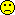## Flawed evaluation accepted [RSABE / ABEL]

Hi Mikalai,

» The identical decision tree was used in the BS of rasagiline (registration required) and was accepted by EMA. https://clinicaldata.ema.europa.eu/web/cdp/home

I succeeded at the end and you are correct. Why am I not surprised that the study was performed in India? OK, the sponsor was European. One of my clients – should have asked me before…Clinical phase ended Jun 2009, study report v1 Oct 2009, v2 Mar 2011, v3 (final) Jul 2011. What happened in those 21 (!) months – waiting for the BE GL (published Jan 2010, effective Jul 2010)?

The procedure for Cmax was almost like Elena described.
• Assess for ABE. If it fails, ABEL will be assessed if both conditions are met:
• PE within 80.00–125.00%,
• CVwR >30% and not the result of outliers.
Interesting:
• PROC MIXED was used with sequence, period, and treatment as fixed effects and subjects as a random effect.
I like that the stupid nested structure subject(sequence) was not used. However, when it comes to ABE, according to the GL all effects fixed (PROC GLM) are preferred.
• Post hoc power to detect a 20% difference under the Null of no difference. Jesusfuckingchrist!
• Lengthy discussion of significant period effect (almost one page).
• Lund’s test of studentized residuals instead of box plots. Given, though see this post why Lund’s test should not be used – except in 2×2×2 crossovers.
• The formula for the CI is stated as $$CI_{1,2}=(\bar{x}_{test}-\bar{x}_{ref})\cdot t_{\alpha/2,N-2}\cdot \mp \sigma \sqrt{2/N}$$, where $$DF=N-2$$.
Oops!
• The error term is only correct if sequences are balanced, i.e., $$n_1=\ldots=n_i$$ or in case of this 2-sequence 4-period replicate study $$n_1=n_2=N/2$$. In general it is $$\sqrt{1/s\sum_{i=1}^{i=s}1/n_i}$$, where $$s$$ is the number of sequences. Here it did not hurt because the study was balanced (13 subjects in each sequence).
• What hurts are the degrees of freedom; $$N-2$$ is only correct for a balanced 2×2×2 crossover. For a balanced 2-sequence 4-period replicate the degrees of freedom are $$3N-4$$. In this case it means the CI would be wider than necessary (24 dfs instead of 74).
Of course, SAS didn’t give a shit on what is written in the protocol and PROC MIXED came up with 69.5 Satterthwaite’s degrees of freedom and calculated the CI correctly.• The regulatory standardized variation $$\sigma_0$$ is given as $$0.25$$ (that’s the one of the FDA) and not as the EMA’s $$\sigma_0=\sqrt{\log_{e}(0.30^2+1)}=0.2935604\ldots$$ and consequently the switching condition $$\theta_s=\frac{\log_{e}(1.25)}{\sigma_0}=0.8925742\ldots$$ which lead to the FDA’s ‘implied limits’ (which are wider than the EMA’s).
However, in the next section all of the above is ignored and the EMA’s $$\theta_s \approx k=0.760$$ is given for ABEL.
• No upper cap of scaling (according to the GL at CVwR 50%) is given.
In the corresponding EPAR we read:

Statistical methods
Analysis of variance (ANOVA) was performed on the ln-transformed Cmax, AUC0–t and AUC0–∞. The fixed effects sequence, subject nested within sequence, period and treatment were used in the ANOVA model to calculate the pharmacokinetic parameters. The test to reference ratio of geometric LSmeans and the corresponding 90% confidence interval based on the ln-transformed Cmax and AUC0–t data were calculated. The parameter Tmax was analyzed using a non-parametric approach.

Criteria for conclusion of bioequivalence:
Bioequivalence was concluded if the test to reference ratio of geometric LSmeans and the corresponding 90% confidence interval for the Cmax and AUC0–t fell within the acceptance limits of 80.00 to 125.00%. Widening of the acceptance criteria for Cmax was proposed for conclusion of bioequivalence. However, since the results of the bioequivalence study for the pharmacokinetic parameter Cmax were within the normal acceptance criteria of 80–125% (as shown below) widening of the criteria for Cmax was not necessary.

The 90% confidence interval of the test/reference ratio (difference in least square means) derived from the ANOVA of the log-transformed pharmacokinetic parameters AUC0–t and Cmax for rasagiline in plasma was within the 80.00% – 125.00% acceptance range. Therefore the test formulation (███████) is judged to be bioequivalent to the reference product (███████) in healthy adult volunteers under fasting conditions.

“The fixed effects sequence, subject nested within sequence, period and treatment were used in the ANOVA model…”
Cough: Not an ANOVA with all effects fixed but a mixed-effects model with restricted maximum likelihood estimation, no nested structure.

Dif-tor heh smusma 🖖Helmut SchützThe quality of responses received is directly proportional to the quality of the question asked. 🚮
Science QuotesIng. Helmut Schütz